Скачать презентацию Covering Non-uniform Hypergraphs Endre Boros Yair Caro Zoltán

a2db0f9ed2586001ffb40c09b228d8e4.ppt

• Количество слайдов: 15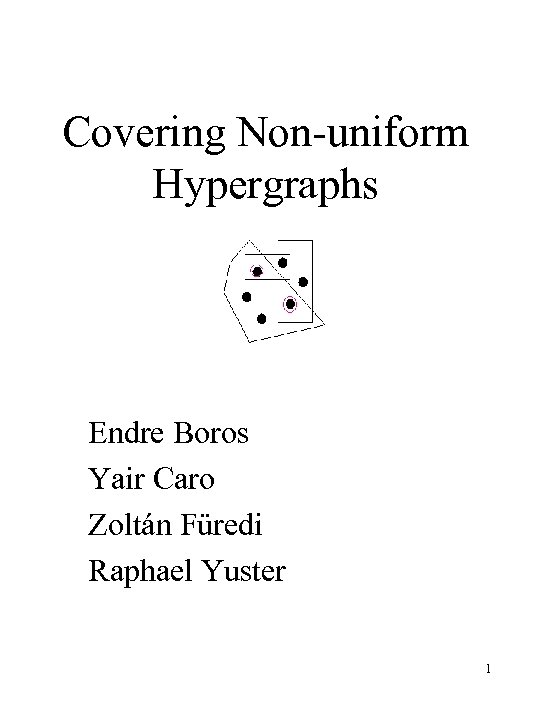Covering Non-uniform Hypergraphs Endre Boros Yair Caro Zoltán Füredi Raphael Yuster 1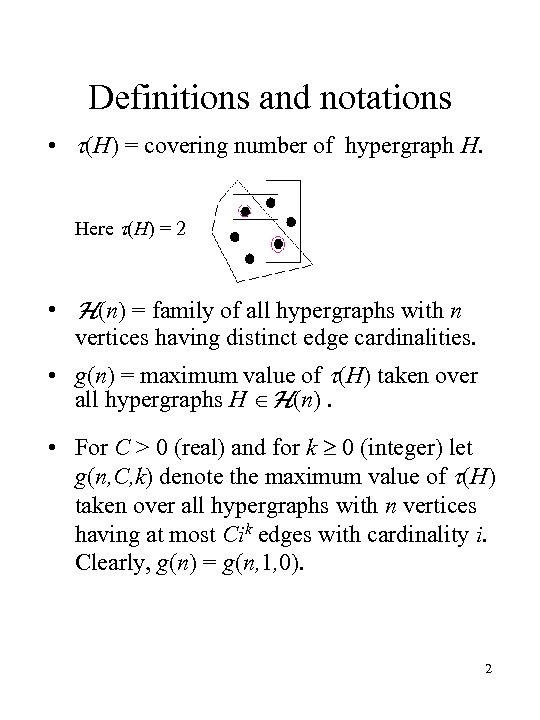Definitions and notations • (H) = covering number of hypergraph H. Here (H) = 2 • H(n) = family of all hypergraphs with n vertices having distinct edge cardinalities. • g(n) = maximum value of (H) taken over all hypergraphs H H(n). • For C > 0 (real) and for k 0 (integer) let g(n, C, k) denote the maximum value of (H) taken over all hypergraphs with n vertices having at most Cik edges with cardinality i. Clearly, g(n) = g(n, 1, 0). 2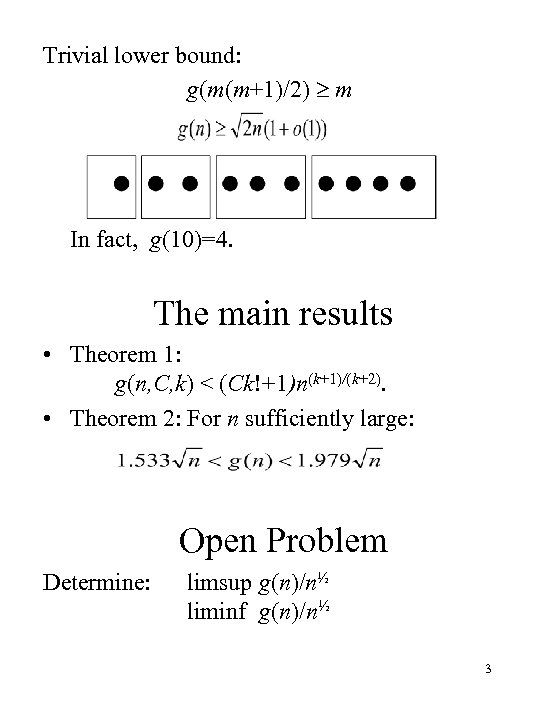Trivial lower bound: g(m(m+1)/2) m In fact, g(10)=4. The main results • Theorem 1: g(n, C, k) < (Ck!+1)n(k+1)/(k+2). • Theorem 2: For n sufficiently large: Open Problem Determine: limsup g(n)/n½ liminf g(n)/n½ 3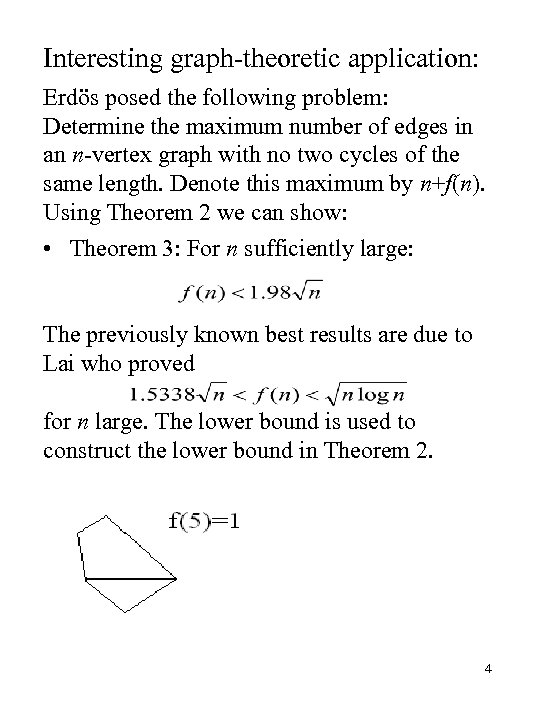Interesting graph-theoretic application: Erdös posed the following problem: Determine the maximum number of edges in an n-vertex graph with no two cycles of the same length. Denote this maximum by n+f(n). Using Theorem 2 we can show: • Theorem 3: For n sufficiently large: The previously known best results are due to Lai who proved for n large. The lower bound is used to construct the lower bound in Theorem 2. 4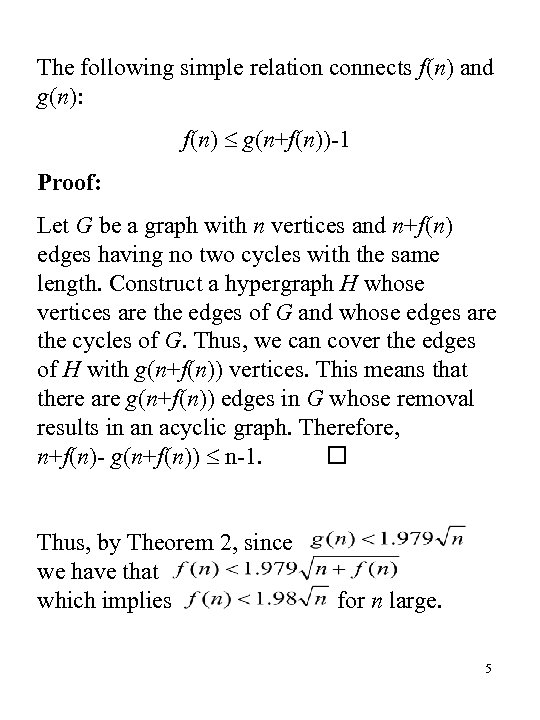The following simple relation connects f(n) and g(n): f(n) g(n+f(n))-1 Proof: Let G be a graph with n vertices and n+f(n) edges having no two cycles with the same length. Construct a hypergraph H whose vertices are the edges of G and whose edges are the cycles of G. Thus, we can cover the edges of H with g(n+f(n)) vertices. This means that there are g(n+f(n)) edges in G whose removal results in an acyclic graph. Therefore, n+f(n)- g(n+f(n)) n-1. Thus, by Theorem 2, since we have that which implies for n large. 5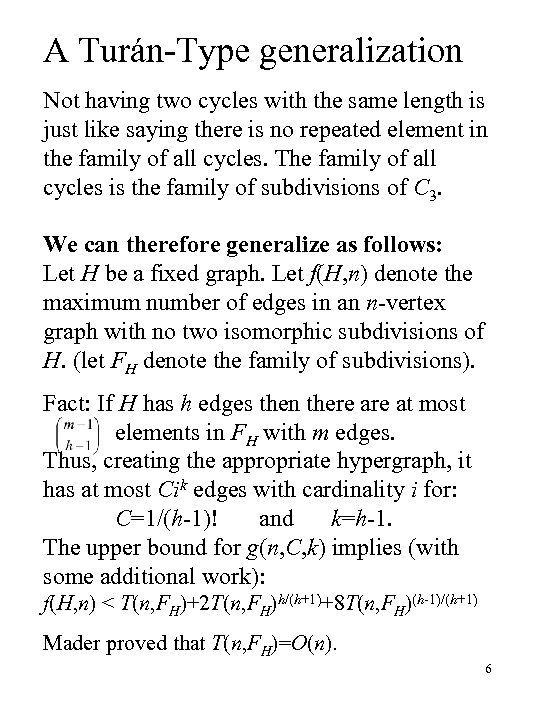A Turán-Type generalization Not having two cycles with the same length is just like saying there is no repeated element in the family of all cycles. The family of all cycles is the family of subdivisions of C 3. We can therefore generalize as follows: Let H be a fixed graph. Let f(H, n) denote the maximum number of edges in an n-vertex graph with no two isomorphic subdivisions of H. (let FH denote the family of subdivisions). Fact: If H has h edges then there at most elements in FH with m edges. Thus, creating the appropriate hypergraph, it has at most Cik edges with cardinality i for: C=1/(h-1)! and k=h-1. The upper bound for g(n, C, k) implies (with some additional work): f(H, n) < T(n, FH)+2 T(n, FH)h/(h+1)+8 T(n, FH)(h-1)/(h+1) Mader proved that T(n, FH)=O(n). 6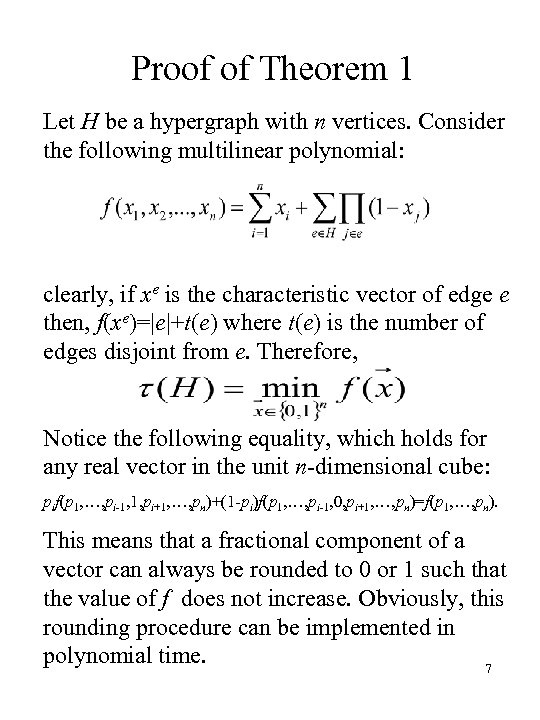Proof of Theorem 1 Let H be a hypergraph with n vertices. Consider the following multilinear polynomial: clearly, if xe is the characteristic vector of edge e then, f(xe)=|e|+t(e) where t(e) is the number of edges disjoint from e. Therefore, Notice the following equality, which holds for any real vector in the unit n-dimensional cube: pif(p 1, …, pi-1, 1, pi+1, …, pn)+(1 -pi)f(p 1, …, pi-1, 0, pi+1, …, pn)=f(p 1, …, pn). This means that a fractional component of a vector can always be rounded to 0 or 1 such that the value of f does not increase. Obviously, this rounding procedure can be implemented in polynomial time. 7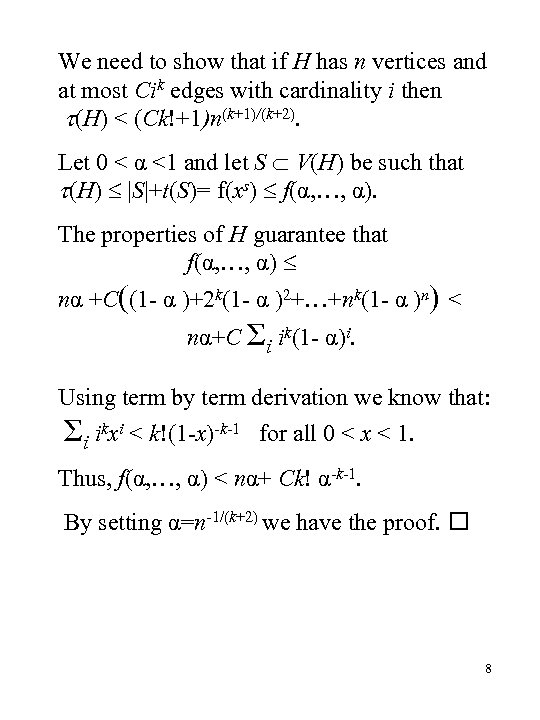We need to show that if H has n vertices and at most Cik edges with cardinality i then (H) < (Ck!+1)n(k+1)/(k+2). Let 0 < α <1 and let S V(H) be such that (H) |S|+t(S)= f(xs) f(α, …, α). The properties of H guarantee that f(α, …, α) nα +C((1 - α )+2 k(1 - α )2+…+nk(1 - α )n) < nα+C Σi ik(1 - α)i. Using term by term derivation we know that: Σi ikxi < k!(1 -x)-k-1 for all 0 < x < 1. Thus, f(α, …, α) < nα+ Ck! α-k-1. By setting α=n-1/(k+2) we have the proof. 8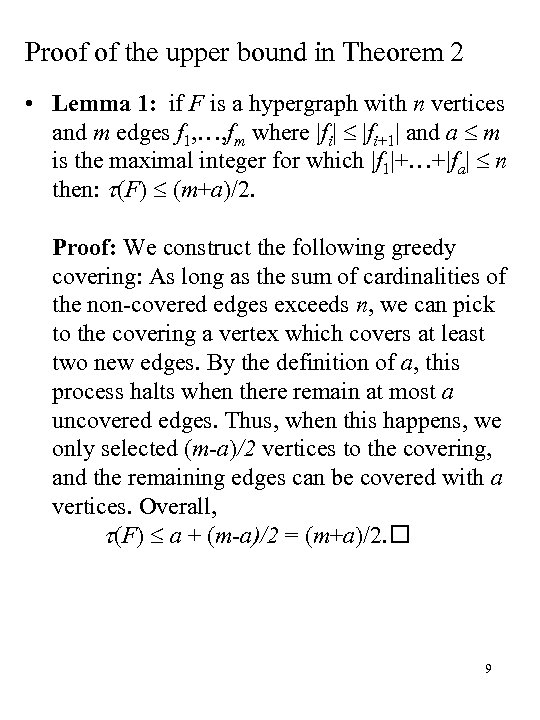Proof of the upper bound in Theorem 2 • Lemma 1: if F is a hypergraph with n vertices and m edges f 1, …, fm where |fi| |fi+1| and a m is the maximal integer for which |f 1|+…+|fa| n then: (F) (m+a)/2. Proof: We construct the following greedy covering: As long as the sum of cardinalities of the non-covered edges exceeds n, we can pick to the covering a vertex which covers at least two new edges. By the definition of a, this process halts when there remain at most a uncovered edges. Thus, when this happens, we only selected (m-a)/2 vertices to the covering, and the remaining edges can be covered with a vertices. Overall, (F) a + (m-a)/2 = (m+a)/2. 9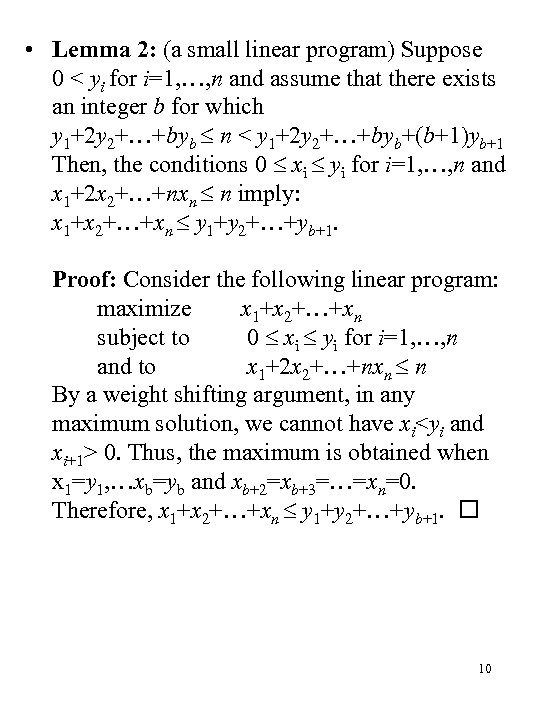• Lemma 2: (a small linear program) Suppose 0 < yi for i=1, …, n and assume that there exists an integer b for which y 1+2 y 2+…+byb n < y 1+2 y 2+…+byb+(b+1)yb+1 Then, the conditions 0 xi yi for i=1, …, n and x 1+2 x 2+…+nxn n imply: x 1+x 2+…+xn y 1+y 2+…+yb+1. Proof: Consider the following linear program: maximize x 1+x 2+…+xn subject to 0 xi yi for i=1, …, n and to x 1+2 x 2+…+nxn n By a weight shifting argument, in any maximum solution, we cannot have xi 0. Thus, the maximum is obtained when x 1=y 1, …xb=yb and xb+2=xb+3=…=xn=0. Therefore, x 1+x 2+…+xn y 1+y 2+…+yb+1. 10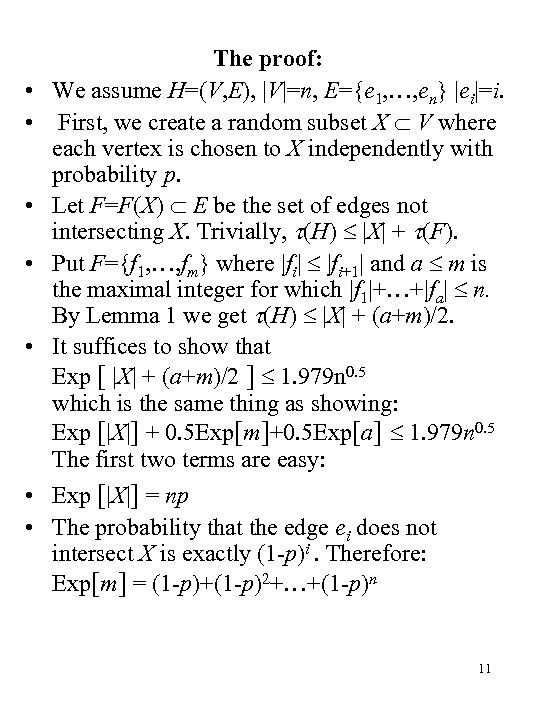• • • The proof: We assume H=(V, E), |V|=n, E={e 1, …, en} |ei|=i. First, we create a random subset X V where each vertex is chosen to X independently with probability p. Let F=F(X) E be the set of edges not intersecting X. Trivially, (H) |X| + (F). Put F={f 1, …, fm} where |fi| |fi+1| and a m is the maximal integer for which |f 1|+…+|fa| n. By Lemma 1 we get (H) |X| + (a+m)/2. It suffices to show that Exp [ |X| + (a+m)/2 ] 1. 979 n 0. 5 which is the same thing as showing: Exp [|X|] + 0. 5 Exp[m]+0. 5 Exp[a] 1. 979 n 0. 5 The first two terms are easy: • Exp [|X|] = np • The probability that the edge ei does not intersect X is exactly (1 -p)i. Therefore: Exp[m] = (1 -p)+(1 -p)2+…+(1 -p)n 11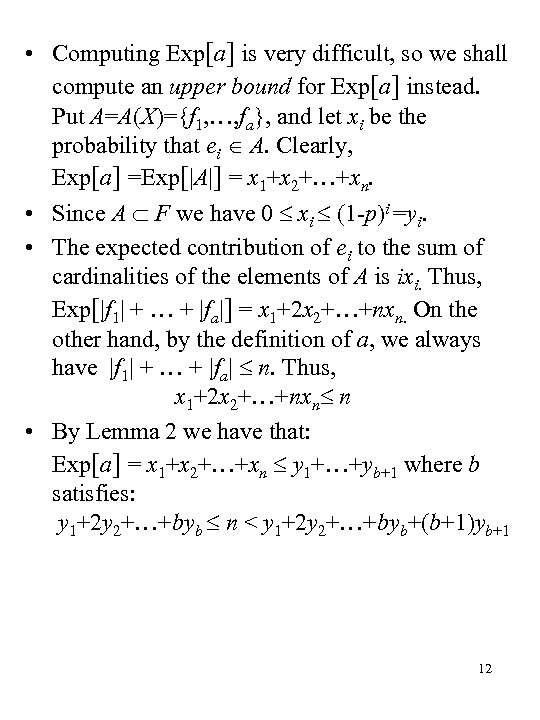• Computing Exp[a] is very difficult, so we shall compute an upper bound for Exp[a] instead. Put A=A(X)={f 1, …, fa}, and let xi be the probability that ei A. Clearly, Exp[a] =Exp[|A|] = x 1+x 2+…+xn. • Since A F we have 0 xi (1 -p)i =yi. • The expected contribution of ei to the sum of cardinalities of the elements of A is ixi. Thus, Exp[|f 1| + … + |fa|] = x 1+2 x 2+…+nxn. On the other hand, by the definition of a, we always have |f 1| + … + |fa| n. Thus, x 1+2 x 2+…+nxn n • By Lemma 2 we have that: Exp[a] = x 1+x 2+…+xn y 1+…+yb+1 where b satisfies: y 1+2 y 2+…+byb n < y 1+2 y 2+…+byb+(b+1)yb+1 12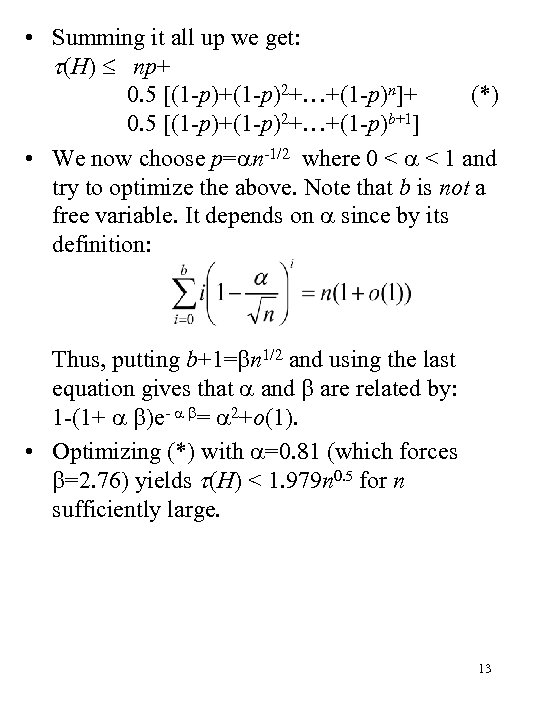• Summing it all up we get: (H) np+ 0. 5 [(1 -p)+(1 -p)2+…+(1 -p)n]+ (*) 0. 5 [(1 -p)+(1 -p)2+…+(1 -p)b+1] • We now choose p= n-1/2 where 0 < < 1 and try to optimize the above. Note that b is not a free variable. It depends on since by its definition: Thus, putting b+1= n 1/2 and using the last equation gives that and are related by: 1 -(1+ )e- = 2+o(1). • Optimizing (*) with =0. 81 (which forces =2. 76) yields (H) < 1. 979 n 0. 5 for n sufficiently large. 13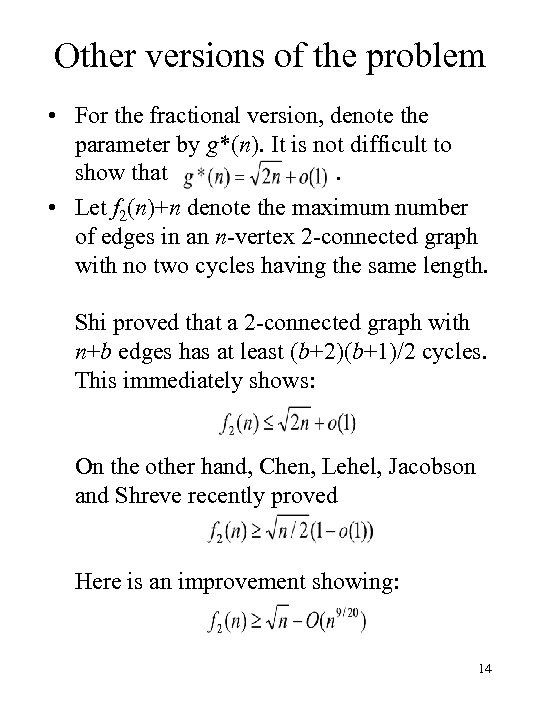Other versions of the problem • For the fractional version, denote the parameter by g*(n). It is not difficult to show that. • Let f 2(n)+n denote the maximum number of edges in an n-vertex 2 -connected graph with no two cycles having the same length. Shi proved that a 2 -connected graph with n+b edges has at least (b+2)(b+1)/2 cycles. This immediately shows: On the other hand, Chen, Lehel, Jacobson and Shreve recently proved Here is an improvement showing: 14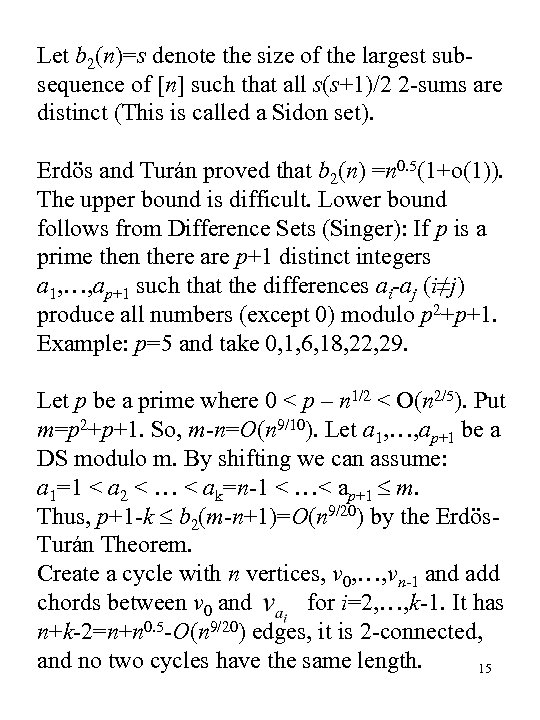Let b 2(n)=s denote the size of the largest subsequence of [n] such that all s(s+1)/2 2 -sums are distinct (This is called a Sidon set). Erdös and Turán proved that b 2(n) =n 0. 5(1+o(1)). The upper bound is difficult. Lower bound follows from Difference Sets (Singer): If p is a prime then there are p+1 distinct integers a 1, …, ap+1 such that the differences ai-aj (i≠j) produce all numbers (except 0) modulo p 2+p+1. Example: p=5 and take 0, 1, 6, 18, 22, 29. Let p be a prime where 0 < p – n 1/2 < O(n 2/5). Put m=p 2+p+1. So, m-n=O(n 9/10). Let a 1, …, ap+1 be a DS modulo m. By shifting we can assume: a 1=1 < a 2 < … < ak=n-1 < …< ap+1 m. Thus, p+1 -k b 2(m-n+1)=O(n 9/20) by the Erdös. Turán Theorem. Create a cycle with n vertices, v 0, …, vn-1 and add chords between v 0 and for i=2, …, k-1. It has n+k-2=n+n 0. 5 -O(n 9/20) edges, it is 2 -connected, and no two cycles have the same length. 15